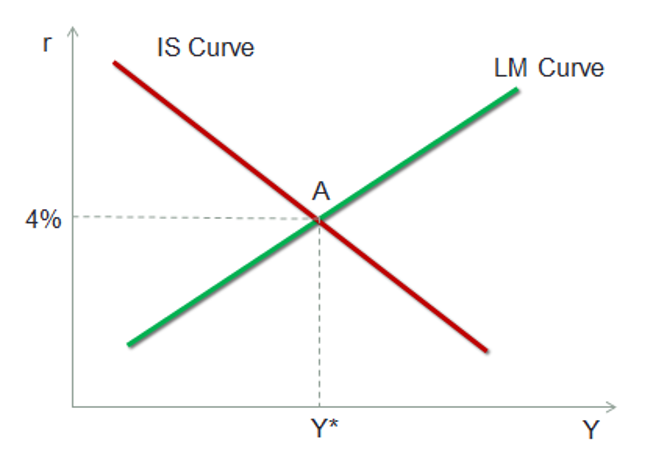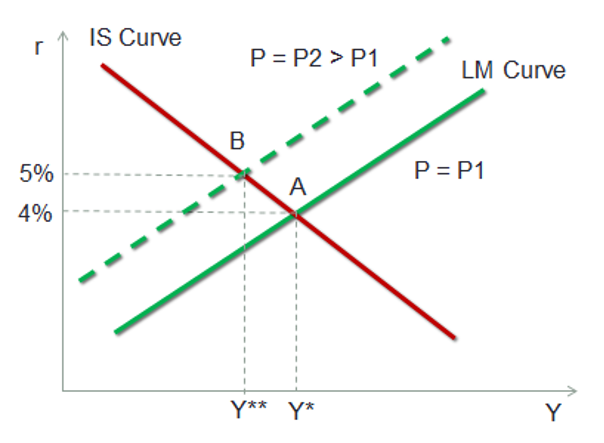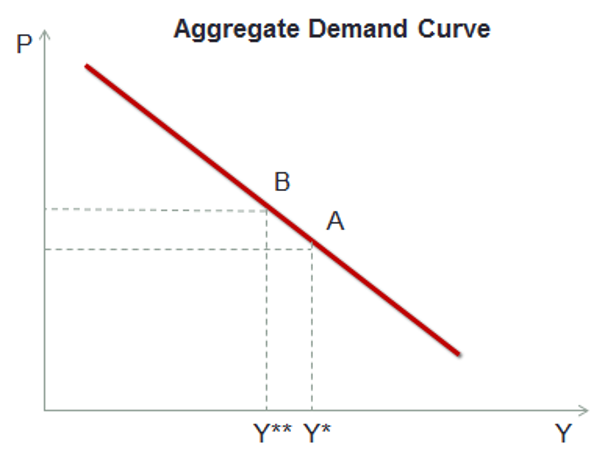# Aggregate Demand Curve

We can use the IS curve and the LM curve to derive the aggregate demand curve. The following curve shows the IS curve and the LM curve with equilibrium at point A where IS intersects with LM. We have assumed that at this equilibrium point the real interest rates are 4%.Let’s assume that at equilibrium level A, the price levels are P1. What will be the impact of increase in price levels to P2? If price levels rise, the real money supply will decrease. Recall that real money supply M/P will decrease if P increases. This will result in a shift of the LM curve to the left. The new equilibrium point will now be B, with a higher real interest rate level and a lower income level, as shown in the graph below.The aggregate demand curve shows the relationship between the quantity of real output demanded (Same as real income) and the price levels. In the above graph we can observe this relationship, i.e., when price levels go up, the real income goes down. The prices and income levels are inversely proportional to each other, as shown in the graph below: